# php导入excel的图片

## 准备工作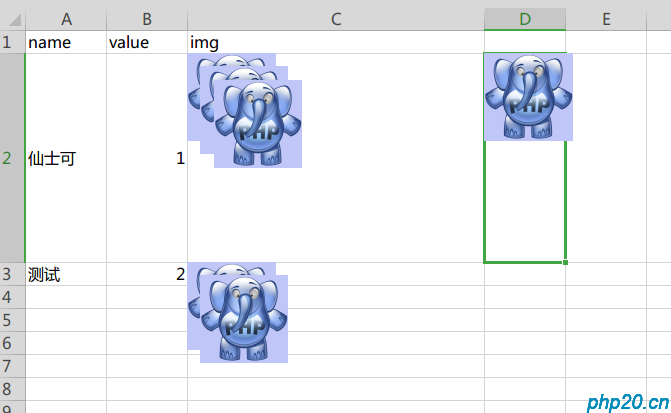## phpexcel库导入

``````composer require PHPOffice/Phpexcel
``````

``````<?php

//获取xlsx文件数据
function getExcel(\$file)
{
\$resule = \$reader->getSheet(0)->toArray(null, false, false, true);//获取首个工作簿信息并转为数组
//过滤空白行
foreach (\$resule as \$key => \$vo) {
if (count(array_unique(\$vo)) == 1) {
unset(\$resule[\$key]);
}
}
array_walk_recursive(\$resule, function (&\$nod) {
\$nod === null ? (\$nod = '') : \$nod = htmlentities(\$nod);
});//NULL转空白字符|拦截XSS

return \$resule;
}

var_dump(getExcel('test.xlsx'));
``````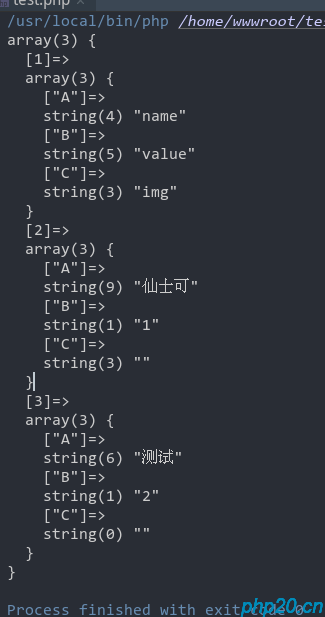### 单独读取表格图片代码:

``````<?php

//获取表格图片
function getImg(\$file)
{
\$sheet = \$excel->getSheet(0);//选择第几个表，如下面图片，默认有三个表
/*取图片*/
\$imgData = array();
\$imageFilePath = 'temp/';//图片保存目录
//遍历表格图片
foreach (\$sheet->getDrawingCollection() as \$img) {
list(\$startColumn,\$startRow)= PHPExcel_Cell::coordinateFromString(\$img->getCoordinates());//获取图片所在行和列

/*表格解析后图片会以资源形式保存在对象中，可以通过getImageResource函数直接获取图片资源然后写入本地文件中*/
//随机一个文件名,用于保存图片
\$imageFileName =  md5(uniqid());
@mkdir(\$imageFilePath,0777,true);
\$imgFile = \$imageFilePath.\$imageFileName.'.'.\$img->getExtension();
copy(\$img->getPath(),\$imgFile);
\$imgData[\$startRow][\$startColumn][] = \$imgFile;//追加到数组中去
}
return \$imgData;
}

var_dump(getImg('test.xlsx'));
``````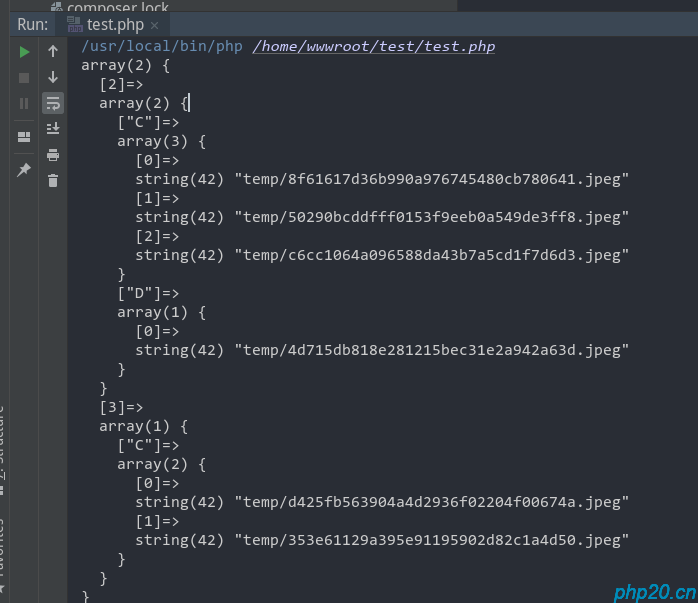##### 热门推荐
•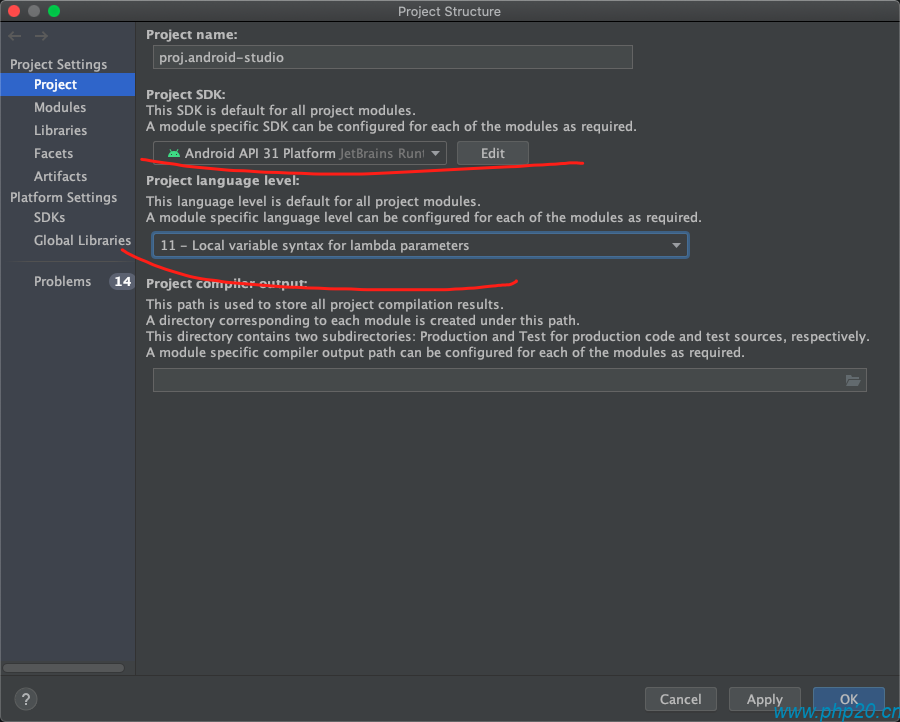浏览(2,407)
•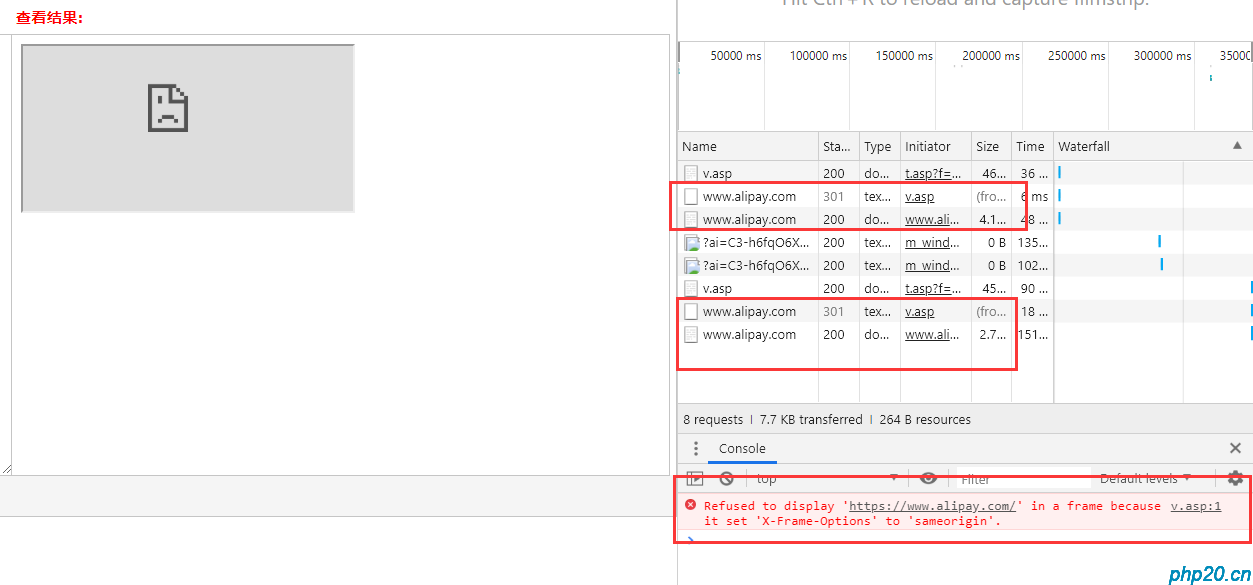浏览(1,735)
••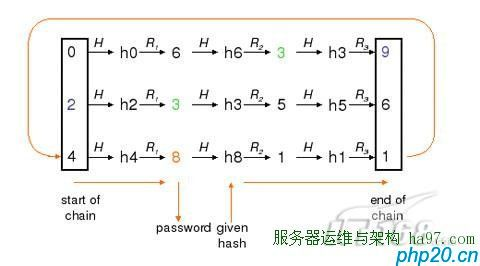•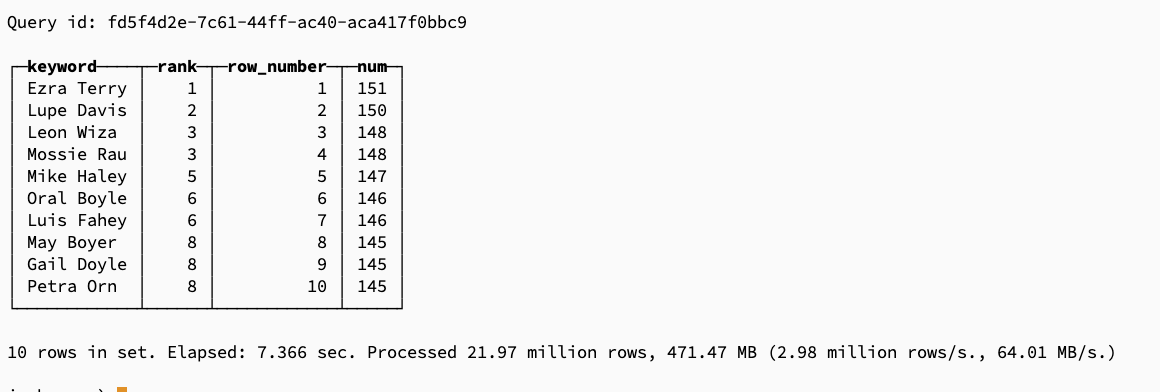浏览(1,232)
•浏览(1,177)
•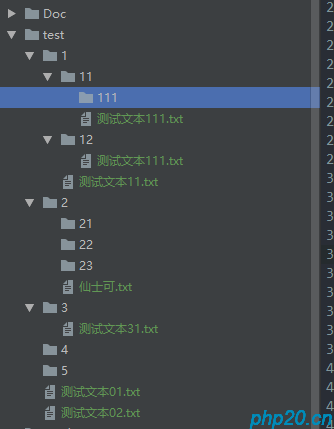浏览(1,160)
•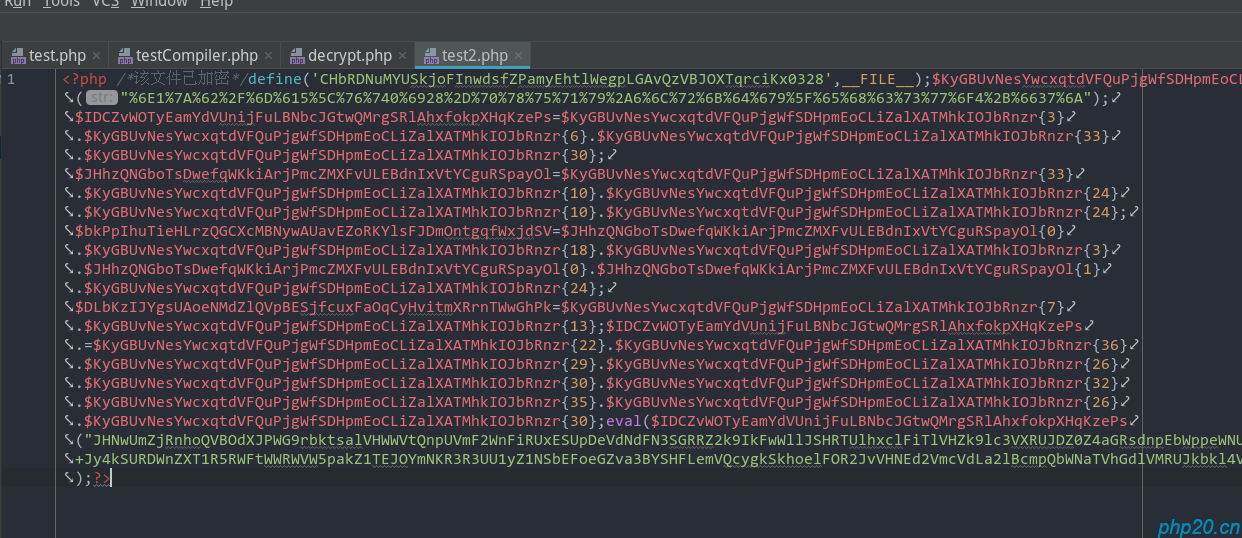浏览(1,150)
•浏览(1,096)
•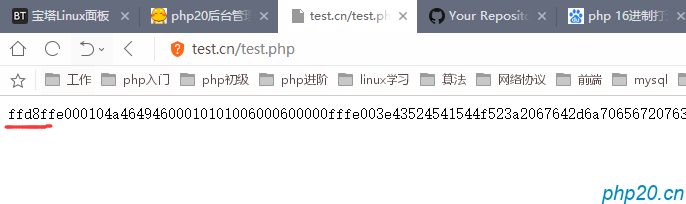浏览(1,070)